### Easter Multiplication and Division Color By Number Fun for Your Students!

Getting students to practice their facts can be challenging, but they will love the fun in coloring these Easter Eggs and you will love the no prep, print and go ease of these Multiplication and Division Color By Number Easter Egg Fun worksheets that include TEN pages for introducing or reviewing multiplication and division by focusing only on multiplication and division facts.Multiplication and Division Color By Number Easter Egg Fun

Multiplication and division are an essential skill to master in third grade. Students need to be able to Interpret products of whole numbers, e.g., interpret 5 × 7 as the total number of objects in 5 groups of 7 objects each.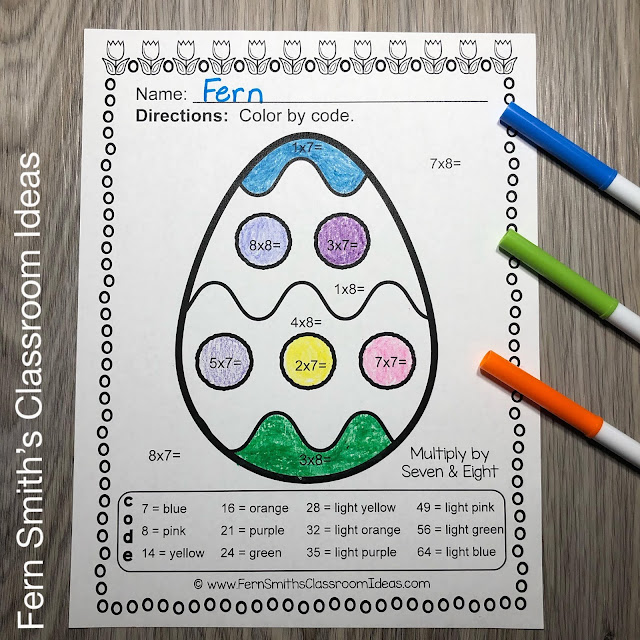Your students will adore their finished pages with these ten adorable Multiplication and Division Color By Number Easter Multiplication and Division worksheets in your classroom while having some Easter Fun learning and reviewing important skills at the same time!Multiplication and Division Color By Number Easter Egg Fun

*** With the 10 Answer Keys also included, this helps you quickly and efficiently check your students work in a very short amount of time. ***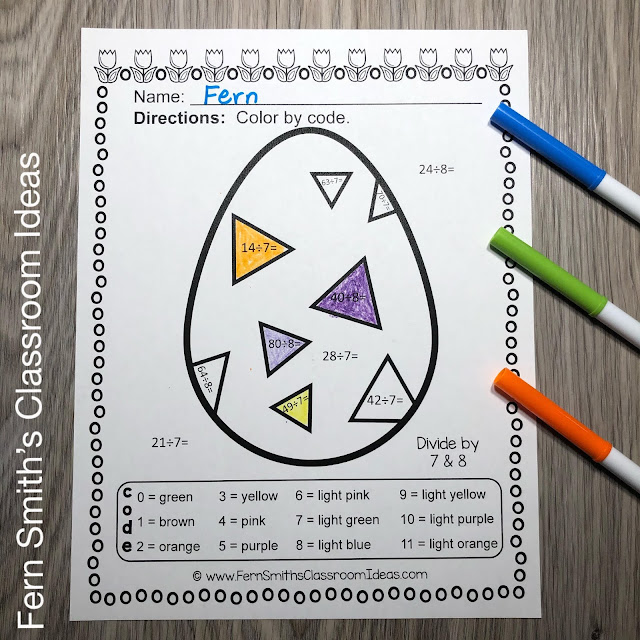Multiplication and Division Color By Number Easter Egg Fun
Multiplication and Division Color By Number Easter Egg Fun

This Easter Multiplication and Division Color By Number math resource includes:

** Each page focuses on a different multiplication and division skill. **

Page One - Multiply by One and Multiply by Two multiplication problems only, (times by 1 & times by 2.)

Page Two - Multiply by Three and Multiply by Four multiplication problems only, (times by 3 & times by 4.)

Page Three - Multiply by Five and Multiply by Six multiplication problems only, (times by 5 & times by 6.)

Page Four - Multiply by Seven and Multiply by Eight multiplication problems only, (times by 7 & times by 8.)

Page Five - Multiply by Nine and Multiply by Ten multiplication problems only, (times by 9 & times by 10.)

Page Six - Divide by One and Divide by Two division problems only, (divide by 1 & divide by 2.)

Page Seven - Divide by Three and Divide by Four division problems only, (divide by 3 & divide by 4.)

Page Eight - Divide by Five and Divide by Six division problems only, (divide by 5 & divide by 6.)

Page Nine - Divide by Seven and Divide by Eight division problems only, (divide by 7 & divide by 8.)

Page Ten - Divide by Nine and Divide by Ten division problems only, (divide by 9 & divide by 10.)

Pages Eleven to Twenty - Answer Keys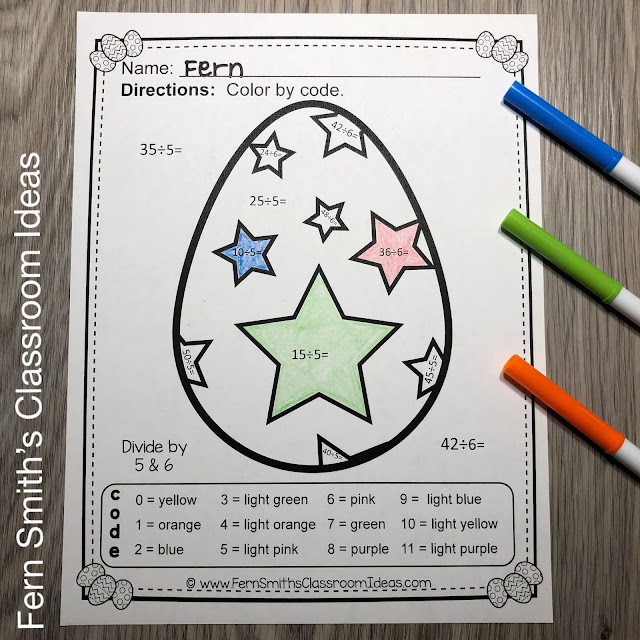Lesson Plan Ideas:

Monday - Use page 1 as an informal assessment, simple and easy with multiplication of 1 and 2 only. OR Use this page to introduce Color By Number to your class with a step by step direct teacher-led lesson on the overhead document camera. Once your students know your procedures, the remaining pages can be used by your students independently. An alternative, based on the ability level of your class is that this teacher-led lesson can also be completed during your math small group lesson on Monday.

Tuesday - Use page 2, during math seat work, math centers or small group time.

Wednesday - Use page 3, during math seat work, math centers or small group time.

Thursday - Use page 4, during math seat work, math centers or small group time. You can also use page 4 as a homework review.

Friday - Use page 5 as a final formal assessment or informal assessment FOR MULTIPLICATION. OR Continue as normal by using page 5 during math seat work, math centers or small group time.

Monday - Use page 6 as an informal assessment, simple and easy with division of 1 and 2 only. OR Based on the ability level of your class is that this teacher-led lesson can also be completed during your math small group lesson on Monday.

Tuesday - Use page 7, during math seat work, math centers or small group time.

Wednesday - Use page 8, during math seat work, math centers or small group time.

Thursday - Use page 9, during math seat work, math centers or small group time. You can also use page 4 as a homework review.

Friday - Use page 10 as a final formal assessment or informal assessment FOR DIVISION. OR Continue as normal by using page 10 during math seat work, math centers or small group time.Terrific for an Emergency Sub Tub or Homework!This resource bundle has the following two resources:Easter Color By Number Multiplication and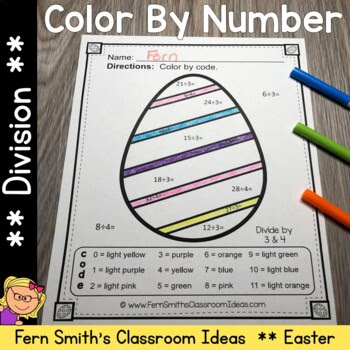Easter Color By Number DivisionTo purchase Color By Number Addition, Subtraction, Multiplication, and Division Bundle Which Includes this Packet, Click Here. Perfect for ELL and ESE classes.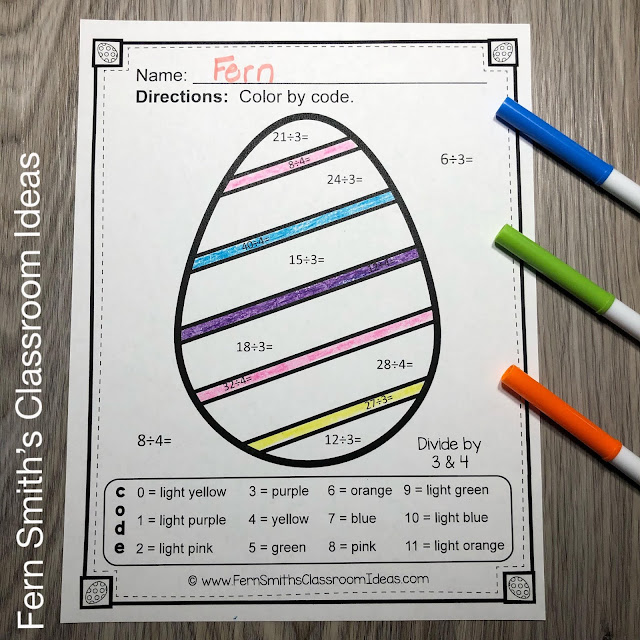These high interest Multiplication and Division Color By Number Easter Multiplication and Division Funky Easter Eggs black and white printables are PERFECT for...

• Remediation and RTI Groups
• Substitute Teacher Folders
• Fine Motor Skills Work
• Small Math Group Lessons
• Math Workstations
• Indoor Recess
• Homework Packets
• Morning Work
• Emergency Sub Tubs
• Morning Math Buckets
• Math Centers
• Early Morning Students
• After School Tutoring
• And so much more!

Terrific for an Emergency Sub Tub, Substitute Teacher Folder, or Homework!Standards

CCSS.3.OA.A.1 - Interpret products of whole numbers, e.g., interpret 5 × 7 as the total number of objects in 5 groups of 7 objects each. For example, describe a context in which a total number of objects can be expressed as 5 × 7.

{Thank you image credit to The Old Barn Teaching!}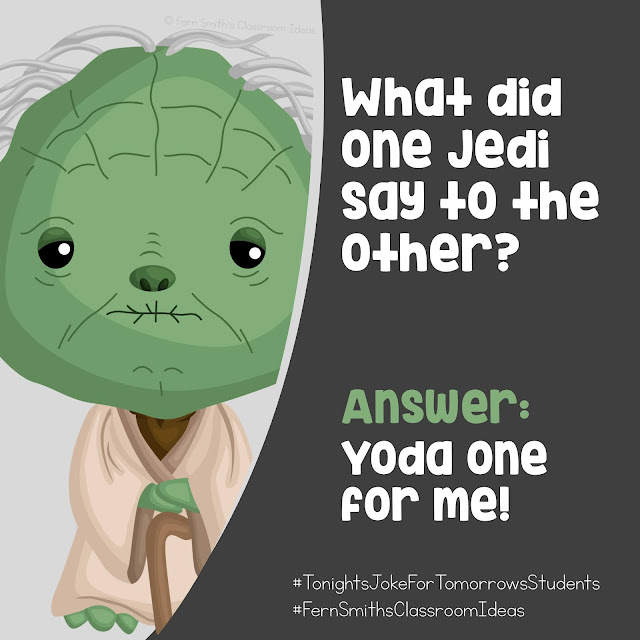𝗧𝗼𝗻𝗶𝗴𝗵𝘁'𝘀 𝗝𝗼𝗸𝗲 𝗳𝗼𝗿 𝗧𝗼𝗺𝗼𝗿𝗿𝗼𝘄'𝘀 𝗦𝘁𝘂𝗱𝗲𝗻𝘁𝘀⁣
What did one Jedi say to the other?⁣
Answer: Yoda one for me! 😂⁣ ⁣
Follow me on Pinterest where I have an entire board dedicated to my jokes for your students and your own personal children! ⁣
⁣⁣ Pinterest: FernSmith ⁣⁣Board: Jokes for Kids. ⁣

Follow Me For More Resources and Freebies!

Don’t forget that all my new products are 40% off for 48 hours.
Click this LINK to follow my shop & you'll be notified of these huge savings.

See ya' tomorrow,